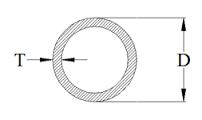# Simultaneously 6272

The first inflow fills the tank in 4 hours. The second tributary in 6 hours. How long will it take to fill both tributaries simultaneously, but the species will start an hour later?

x =  2.8 h

### Step-by-step explanation:

x/4 + (x-1)/6=1

5x = 14

x = 14/5 = 2.8

x = 14/5 = 2.8

Our simple equation calculator calculates it.Did you find an error or inaccuracy? Feel free to write us. Thank you!

Tips for related online calculators
Do you have a linear equation or system of equations and looking for its solution? Or do you have a quadratic equation?
Do you want to convert time units like minutes to seconds?